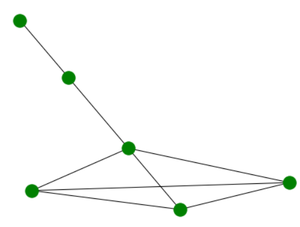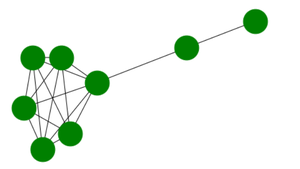Open in App
Not now

# Lollipop Graph in Python using Networkx module

• Last Updated : 29 Apr, 2021

The lollipop graph consists of 2 components a complete graph called clique and a path graph. More precisely L (m ,n)  is a graph with an m-node complete graph and n-node path graph.

L(4,2) Lollipop Graph:L(4,2) Graph

Here let’s see the properties of this graph:

We will use the networkx module for realizing a Lollipop graph. It comes with an inbuilt function networkx.lollipop_graph() and can be illustrated using the networkx.draw() method. This module in Python is used for visualizing and analyzing different kinds of graphs.

Syntax: networkx.lollipop_graph(m,n)

Parameters:

• M: Number of nodes in complete graph(clique)
• N: Number of nodes in path graph.

networkx.draw(G, node_size, node_color)

• Used to realize the graph by passing graph object.
• G: It refers to the Tutte graph object
• node_size: It refers to the size of nodes.
• node_color: It refers to color of the nodes.

Example 1: Create a simple lollipop graph

Approach:

• We will import the required module network
• Then we will create a graph object using networkx.lollipop_graph(m,n).
• For realizing graph, we will use networkx.draw(G, node_color=’green’)
• With three arguments first one being graph object and the other two used to set the color and size of node.

Code:

## Python3

 `# import required module``import` `networkx` `# create object``G ``=` `networkx.lollipop_graph(``4``, ``2``)` `# illustrate graph``networkx.draw(G, node_color``=``'green'``)`

Output:Example 2: Changing the size of the nodes.

Approach:

• We will import the required module network.
• Then we will create a graph object using networkx.lollipop_graph(m,n).
• For realizing graph, we will use networkx.draw(G, node_color=’green’,node_size=1500)
• Note that here we have passed an extra argument in draw function namely node_size.
• This extra argument determines the relative size of the node.

Code:

## Python3

 `# import required module``import` `networkx` `# create object``G ``=` `networkx.lollipop_graph(``6``, ``2``)` `# illustrate graph``networkx.draw(G, node_color``=``'green'``,``              ``node_size``=``1500``)`

Output:Example 3: Creating Lollipop graph without using lollipop_graph() function.

Approach:

• We will import the required module networkx as nx.
• We will then manually define lollipop_graph function.
• We will first create the complete graph using complete_graph function.
• Then we will n nodes numbered from m to m+n-1 which will be from straight part of lollipop
• Then we will join the adjacent nodes to complete the path graph part of lollipop.
• At last we will join the two disjoint parts to obtain the final result.
• Note that two if conditions are used to check whether the number of nodes in either of the part is needed to be added or that part is to be kept empty.

Code:

## Python3

 `# importing networkx``import` `networkx as nx`  `def` `lollipop_graph(m, n):` `    ``# the complete graph part.``    ``G ``=` `nx.complete_graph(m)` `    ``# adding path graph edges.``    ``G.add_nodes_from([v ``for` `v ``in` `range``(m, m``+``n)])``    ``if` `n > ``1``:` `        ``# joining adjacent path graph edges.``        ``G.add_edges_from([(v, v``+``1``) ``for` `v ``in` `range``(m, m ``+` `n ``-` `1``)])` `    ``# connect complete graph to path graph``    ``if` `m > ``0``:``        ``G.add_edge(m ``-` `1``, m)``    ``return` `G`  `# invoking the function defined.``G ``=` `lollipop_graph(``5``, ``2``)``nx.draw(G)`

Output:My Personal Notes arrow_drop_up